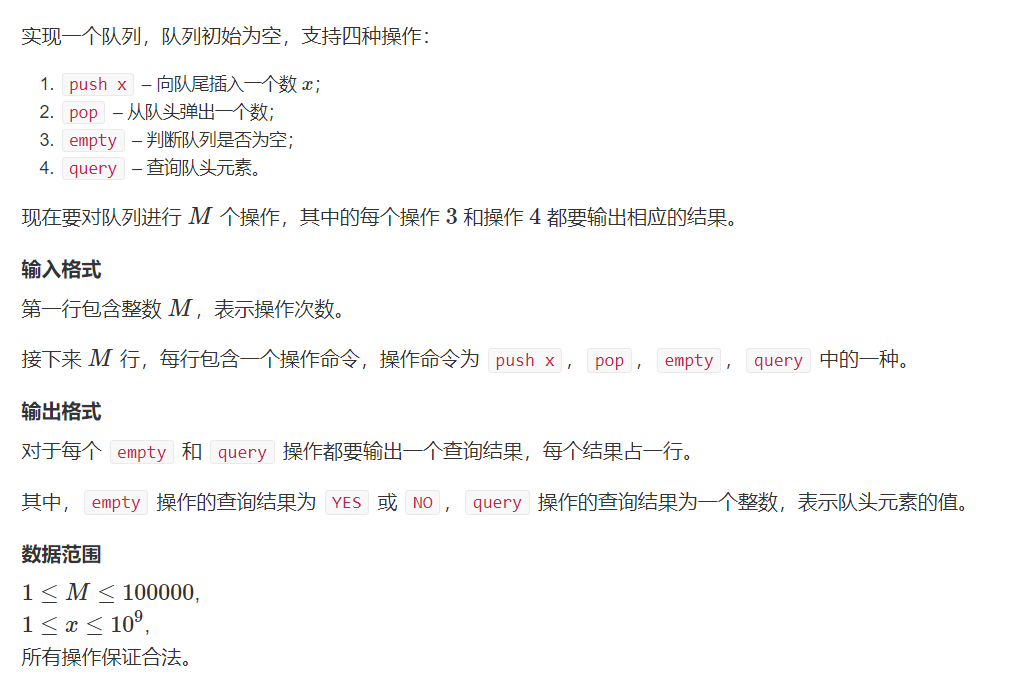> 文档中心 > 数据结构入门——队列

# 关于设置关注可见

### 0.序

while(keep) ability++;

### 队列

#### 概念

• 队列是一个线性的数据结构(应该很好懂)，同时队列是一个受限制的线性数据结构。
• 我们只能在一端添加元素，另一端删除元素(和数组相比实在是太弱了）
• 能添加元素的一端称为队尾，删除元素的一端称为队头
• 这时我们就能发现，这是一个先进先出的结构，就像是排队一样，从尾巴进去 从头上出来hh

• 添加
• 删除
(在上面已经提到了hh)

#### 关于实现一个队列

tail:记录队尾的位置

int head = 1, tail = 0;int q[100000 + 10];void push(int x) {q[++tail] = x;}int pop() {return q[head++];}

queue q;q.push(x);q.pop();

#### 举个栗子#include #include #include const int N = 1000000 + 10;using namespace std;int q[N], head = 1, tail = 0;void push(int x) {    q[++tail] = x;}int pop() {    return q[head++];}int main(){    int n;    cin >> n;    string s;    for (int i = 1; i <= n; i++) { cin >> s; if (s == "push") {     int x;     cin >> x;     push(x); } else if (s == "pop") {     pop(); } else if (s == "empty") {     if (tail < head) {  cout << "YES" << '\n';     }     else puts("NO"); } else {     cout << q[head] << '\n'; }    }    return 0;}

#### 举个栗子

[1 3 -1] -3 5 3 6 7 -1 3
1 [3 -1 -3] 5 3 6 7 -3 3
1 3 [-1 -3 5] 3 6 7 -3 5
1 3 -1 [-3 5 3] 6 7 -3 5
1 3 -1 -3 [5 3 6] 7 3 6
1 3 -1 -3 5 [3 6 7] 3 7

##### 输入样例：
8 31 3 -1 -3 5 3 6 7
##### 输出样例：
-1 -3 -3 -3 3 33 3 5 5 6 7

#### 怎么搞一个单调队列

#include #include #include const int N = 1000000 + 10;using namespace std;int n, k, a[N];int q[N], head = 1, tail = 0;int p[N];void print_min() {head = 1;tail = 0;for (int i = 1; i <= n; i++) {while (head = a[i]) {tail--;}q[++tail] = a[i];p[tail] = i;while (p[head] = k) {printf("%d ", q[head]);}}puts("");}void print_max() {head = 1;tail = 0;for (int i = 1; i <= n; i++) {while (head <= tail && q[tail] <= a[i]) {tail--;}q[++tail] = a[i];p[tail] = i;while (p[head] = k) {printf("%d ", q[head]);}}puts("");}int main(){    cin >> n >> k;    for (int i = 1; i > a[i];    }    print_min();    print_max();    return 0;}